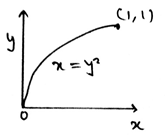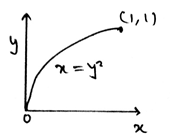Courses

# Test: Centre Of Gravity

## 15 Questions MCQ Test Engineering Mechanics | Test: Centre Of Gravity

Description
This mock test of Test: Centre Of Gravity for Mechanical Engineering helps you for every Mechanical Engineering entrance exam. This contains 15 Multiple Choice Questions for Mechanical Engineering Test: Centre Of Gravity (mcq) to study with solutions a complete question bank. The solved questions answers in this Test: Centre Of Gravity quiz give you a good mix of easy questions and tough questions. Mechanical Engineering students definitely take this Test: Centre Of Gravity exercise for a better result in the exam. You can find other Test: Centre Of Gravity extra questions, long questions & short questions for Mechanical Engineering on EduRev as well by searching above.
QUESTION: 1

### A body is consisted of __________ number of particles.

Solution:

The various bodies are composed of infinite number of particles. They are very small in size. They cant be seen by our naked eyes. Thus the number of particles is not thousands nor hundreds. But it is uncountable.

QUESTION: 2

### A body’s all small particles have a small weight which is being applied by them to the body, which adds up to the total weight of the body.

Solution:

The weight of the body is the sum of the all the small weights that are been applied by all the particles. The smaller the particle the smaller the weight it applies. Thus the total weight of the body is the summation of all the infinite number of small weights applied to the body.

QUESTION: 3

### The all small weights that are being applied by all the infinite particles of the body act __________ to each other.

Solution:

All the small weights that are being applied by all the particles are being applied by them parallel to themselves. Thus the parallel is the right answer. Thus the total sum of all these small weights add up to the total weight of the body.

QUESTION: 4

The total of all the weights of small particles adds up to give the total body weight. This weight is the force vector which is being passed by ________

Solution:

The total body weight always passes through the centre of gravity. All the forces are parallel is the right answer. Thus the total sum of all these small weights add up to the total weight of the body. Which passes through the centre of gravity.

QUESTION: 5

The centre of gravity is the ratio of ________ to _________

Solution:

The product of the centroid of the section to its weight to the total weight of the body is the centre of gravity. Thus the answer. The ratio is generally used to locate the coordinate of the centroid or the centre of gravity.

QUESTION: 6

The x axis coordinate and the y axis coordinate of the centre of gravity are having different types of calculations to calculate them.

Solution:

The calculation is general to all the axis of coordinate system. This means that the calculations are generalised for all the axis. And it is the product of the centroid of the section to its mass to the total weight of the body is the centre of gravity.

QUESTION: 7

The centre of mass is the ratio of ________ to _________

Solution:

The product of the centroid of the section to its mass to the total mass of the body is the centre of mass. Thus the answer. The ratio is generally used to locate the coordinate of the centroid or the centre of mass.

QUESTION: 8

The centre of volume is the ratio of ________ to _________

Solution:

The product of the centroid of the section to its volume to the total volume of the body is the centre of volume. Thus the answer. The ratio is generally used to locate the coordinate of the centroid or the centre of volume.

QUESTION: 9

Determine the y coordinate of centroid of the line in the shape of parabola as shown.Solution:

The weight of the body is the sum of the all the small weights that are been applied by all the particles. The smaller the particle the smaller the weight it applies. Thus the total weight of the body is the summation of all the infinite number of small weights applied to the body.

QUESTION: 10

Volume is best given by ___________

Solution:

The density is the ration of the mass to the volume of the body. Thus arranging them and the volume is said to be as ratio of mass to density. This equation is generally used in the calculations of the centroid of the body or the centre of gravity.

QUESTION: 11

We use sometimes the measures to know the direction of moment. It is done by right handed coordinate system. Which is right about it(consider the mentioned axis to be positive)?

Solution:

As right handed coordinate system means that you are curling your fingers from positive x-axis towards y-axis and the thumb which is projected is pointed to the positive z-axis. Thus visualizing the same and knowing the basic members of axis will not create much problem.

QUESTION: 12

If A is any vector with Ai + Bj + Ck then what is the y-axis component of the vector if we are using the vector math to calculate the centre of gravity?

Solution:

As the given vector is shown in the Cartesian system, the number with “j” is the Y-axis component of the given vector. With the numbers with “i” representing components of x-axis and “k” representing the z axis respectively.

QUESTION: 13

If the force vector F acting along the centre of gravity is having its x-axis component being equal to Z N, y-axis component be X N and z-axis component be Y N then vector F is best represented by?

Solution:

It is given that x, y and z-axis components are Z, X and Y respectively. Thus, just by placing the right coordinates we get option d to be correct. Here just the interchange of the axis’s representor with their respective axis is creating confusion. Which must be figured out.

QUESTION: 14

Which statement is right for force acting by the small particles of the body having it’s vector form as = Ai + Bj + Ck?

Solution:

As given the vector is F = Ai + Bj + Ck, this implies that the x ,y and z-axis components of this vector is A, B and C respectively. But, in rectangular components representation of any vector, the vector is written as F = Fx + Fy + Fz.

QUESTION: 15

Determine the x coordinate of centroid of the line in the shape of parabola as shown.Solution:

The weight of the body is the sum of the all the small weights that are been applied by all the particles. The smaller the particle the smaller the weight it applies. Thus the total weight of the body is the summation of all the infinite number of small weights applied to the body.